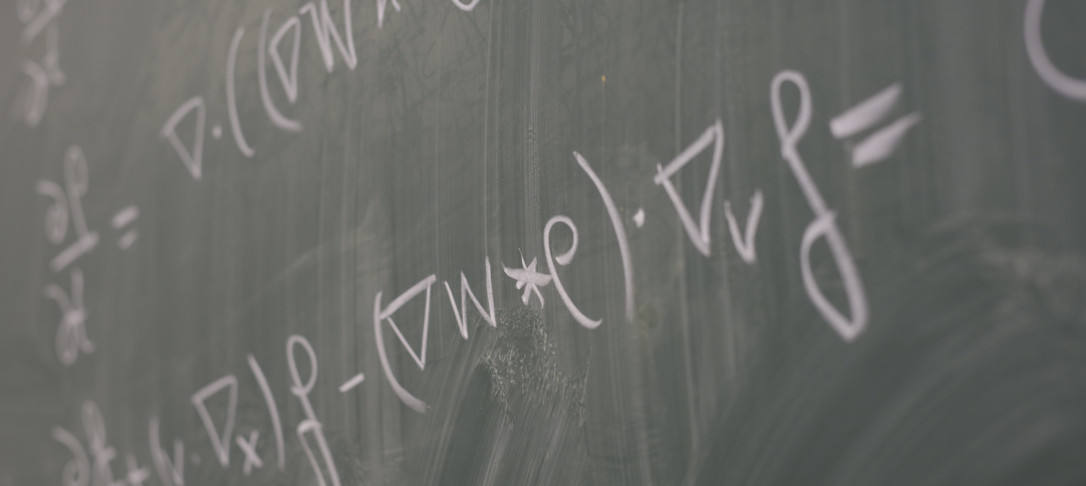## Title

The Geometry of Monge–Ampère Equations in Fluid Dynamics

## Abstract

The Poisson equation for the pressure of a homogeneous, incompressible Navier–Stokes flow is a key diagnostic relation for understanding the formation of vortices in turbulence. Building from the observation that, in two dimensions, the aforementioned equation is a Monge–Ampère equation for the stream function, this talk introduces a framework for studying fluid dynamics using the geometry of such Monge–Ampère equations.

In particular, we show that the associated Lychagin–Rubtsov metric on the phase space of the flow encodes the Laplacian of pressure, while the pull-back of this metric to a Lagrangian submanifold (which represents a solution of the original PDE) has eigenvalues and signature determined by the dominance of vorticity and strain. We highlight how this framework generalises to fluid flows on curved background and to higher dimension, by inferring a Weiss–Okubo criterion for fluid flows on an arbitrary Riemannian manifold. We conclude with some comments on generalised solutions as a model for vortex sheets and shockwaves, the topology of vortices, and some open questions.

Note: This talk is intended as an introduction to Monge–Ampère geometry as a tool for studying PDEs, using Navier–Stokes flows as a case study. While some knowledge of differential geometry would be useful, I do not assume this a-priori, and the talk will be relatively self-contained, with the relevant concepts introduced as we proceed.

Please note that the seminar will take place in person in room 642 of Huxley Building.